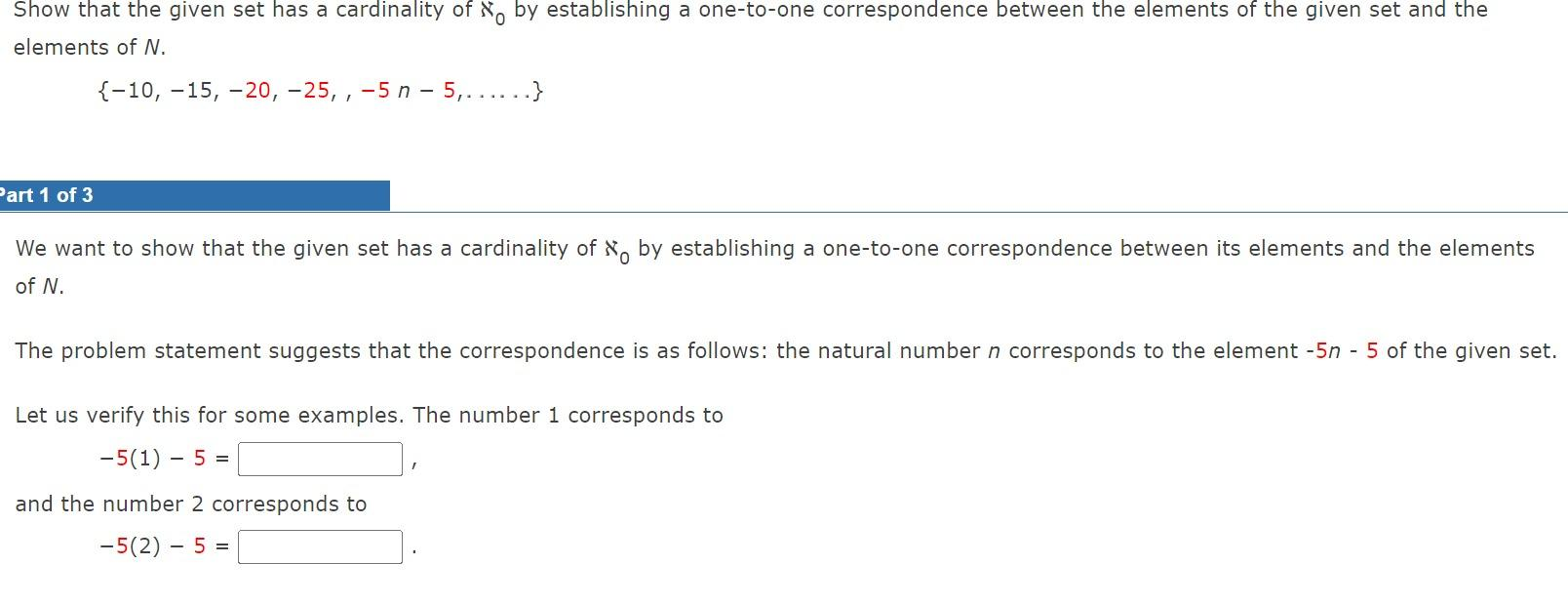Home / Expert Answers / Algebra / show-that-the-given-set-has-a-cardinality-of-aleph-0-by-establishing-a-one-to-one-corresp-pa155

# (Solved): Show that the given set has a cardinality of \( \aleph_{0} \) by establishing a one-to-one corresp ...Show that the given set has a cardinality of \( \aleph_{0} \) by establishing a one-to-one correspondence between the elements of the given set and the elements of \( N \). \[ \{-10,-15,-20,-25,-5 n-5, \ldots \ldots\} \] ? \( \operatorname{art} 1 \) of 3 We want to show that the given set has a cardinality of \( \aleph_{0} \) by establishing a one-to-one correspondence between its elements and the elements of \( N \). The problem statement suggests that the correspondence is as follows: the natural number \( n \) corresponds to the element \( -5 n \) - 5 of the given set. Let us verify this for some examples. The number 1 corresponds to \[ -5(1)-5= \] and the number 2 corresponds to \[ -5(2)-5= \]

We have an Answer from Expert